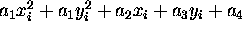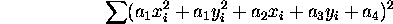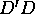Next: About this document Up: Least-square fitting of circles Previous: Approximated Euclidean Distance

## Algebraic Distance

The algebraic distance of a pointto a circle is simply. Thus, we have a simple procedure to estimate the parameters: find the parametersthat minimizesDefine the design matrix:The least square solution is the eigenvector of the smallest eigenvalue of the matrix.

Some matlab code for the fit is here and for conversion to standard form is here.

Bob Fisher
Thu Aug 3 17:26:57 BST 2000